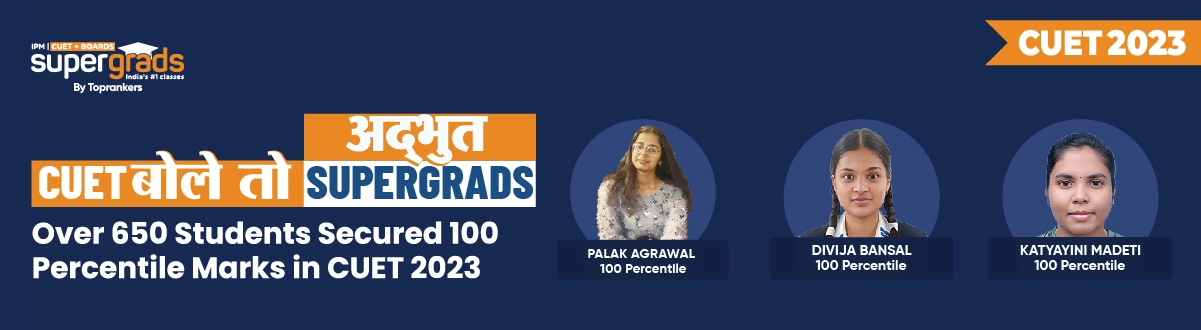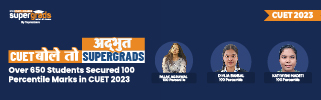# CBSE Class 11 Applied Mathematics 2024: Concepts, Syllabus & Books

Author : Tanya Kaushal

Updated On : November 15, 2023

SHARE

Reader's Digest: Want to know the essentials of CBSE Applied Maths for Class 11? Read this blog to learn about CBSE Class 11 Applied Mathematics, from its core concepts to the latest syllabus updates, recommended books & effective preparation strategies.

As the academic year unfolds, CBSE students across India are gearing up for a new addition to their curriculum - Class 11 Applied Mathematics.

This dynamic subject promises to bridge the gap between theoretical mathematics and its real-world applications, offering students a unique perspective on problem-solving and mathematical modelling.

You must take the exam of 3 hours of total 80 marks. However, 20 marks are allotted for an Internal Assessment.

Here are the key points we will be discussing in this blog:

• Meaning of CBSE Class 12 Applied Mathematics: A brief introduction to the subject and its significance in the modern educational landscape.
• Class 11 Applied Maths Syllabus: A comprehensive syllabus breakdown highlighting the topics and chapters students can expect to study.
• Applications of CBSE Applied Mathematics: Exploring the real-world applications of this subject and how it connects theoretical knowledge to practical scenarios.
• CBSE Class 11 Applied Mathematics Prep Tips: Valuable tips and strategies to excel in this subject, including study techniques and recommended resources.

## What is CBSE Applied Mathematics?

Applied Mathematics combines mathematical science and specialized knowledge in different subjects. This subject extensively uses mathematics techniques in other fields like Physics, Engineering, Medical, and more.

The practical applications of Maths have provided a way to develop Mathematical Theories, resulting in the pure study of mathematics.

CBSE has decided to add this subject to the curriculum to help students understand it in-depth if they choose Maths as their career. Also, Applied Mathematics has various applications explained in the below section.## CBSE Class 11 Applied Mathematics Press Release

As per the Systematic Reforms, the National Curriculum Framework 2005 recommends examining Maths and English at two levels. Hence, CBSE considered this recommendation to introduce Applied Mathematics.

Maths is a widely used subject in every career, which can help a student's life. Also, it is observed that the current Maths syllabus goes well with the Science stream but not with Commerce and other streams. Hence, this subject is an elective course that can help to have a great career ahead.

### Main Objectives of Introducing Applied Mathematics

• To have an interconnection with other subjects.
• To help in developing logical reasoning skills and apply the same in problem-solving.
• To develop an understanding of basic mathematical and statistical tools and their applications in the field of commerce (business/finance/economics) and social sciences;
• To implement real-world experiences/problems into mathematical expressions using numerical/algebraic/graphical representation;
• To make sense of the data by organizing, representing, interpreting, analyzing, and making meaningful inferences from real-world situations;

Read More: CBSE Class 11 Applied Math Books

## CBSE Class 11 Applied Maths Syllabus 2024

In the CBSE Class 11 Applied Mathematics subject, seven chapters are tabulated below. Go through the topic name and marking scheme of the CBSE 11th class Applied Mathematics Syllabus below:

 No. Units No. of Periods Marks I. Numbers, Quantification, and Numerical Applications 25 09 II. Algebra 45 15 III. Mathematical Reasoning 15 06 IV. Calculus 35 10 V. Probability 25 08 VI. Descriptive Statistics 35 12 VII Basics of Financial Mathematics 45 18 VIII Coordinate Geometry 15 05 - Total 240 80 - Internal Assessment - 20

Read More: CBSE Class 11 Applied Maths - Mathematical Reasoning

## What are the Practical Applications of CBSE Class 11 Applied Mathematics?

There is a wide range of applications of Applied Mathematics in various fields. In many streams, Applied Maths plays an important role. Some of the typical applications of CBSE Class 11 Applied Mathematics are listed below:

• Information Theory
• Engineering
• Signal Processing
• Cryptography
• Operations Research
• Numerical Analysis
• Statistics
• Wavelets
• Game Theory

## What are the Best Preparation Tips for CBSE Class 11 Applied Mathematics 2024?

Preparation for any subject varies for each student; however, here are a few experts' suggested tips and tricks that can help enhance your preparation.

• Structured Study Plan: Create a well-structured study plan that covers all the topics. Allocate more time to challenging areas and revise regularly. Stick to your schedule diligently.
• Quality Resources: Choose suitable study materials and resources. Look for reference books, online courses, and video tutorials to supplement your textbooks. Quality matters more than quantity. Refer to the best CBSE Class 11 Commerce Books per the latest pattern and syllabus.
• Practice Regularly: Mathematics is a subject that requires consistent practice. Solve a wide variety of problems to reinforce your understanding. Make use of previous years' question papers and sample papers.
• Conceptual Clarity: Ensure you have a deep understanding of the fundamental concepts. Don't just memorize formulas; understand how and when to apply them.
• Keep Formula Notes: Maintain a separate notebook for essential formulas, theorems, and key concepts. This will be handy for quick revisions.

## Difference Between Core Maths & Applied Maths

One of the most common doubts with all students is the difference between pure and applied maths. Here are the significant differences between Pure Maths and Applied Maths explained.

Aspect Core Mathematics Applied Mathematics
Emphasis Focuses on theoretical concepts and abstract mathematics. Emphasizes practical applications of mathematical concepts.
Content Includes topics like algebra, calculus, geometry, and statistics. Covers topics like financial mathematics, statistics, and optimization.
Theoretical vs. Practical Primarily theoretical, with a focus on proofs and theorems. Primarily practical, with a focus on real-world problem-solving.
Application Areas Generally applicable across various fields and sciences. Specifically designed for practical use in specific fields like business, economics, and social sciences.
Problem-Solving Approach Often involves solving complex mathematical problems and proofs. Involves solving real-world problems and making data-driven decisions.
Mathematical Rigor Emphasizes mathematical rigour and precision in solutions. Focuses on finding practical solutions, often with some degree of approximation.
Relevance in Careers Beneficial for careers in mathematics, physics, engineering, and academia. Useful for finance, economics, data science, and social sciences careers.
Common Courses and Degrees Included in most standard mathematics curricula. Often offered as a specialized course or as part of specific degree programs.
Examples of Topics Calculus, linear algebra, number theory, abstract algebra. Financial mathematics, data analysis, operations research.
Academic Levels Taught at various academic levels, from high school to university. Typically taught at the university or college level.
Problem Complexity Problems can be highly theoretical and abstract. Problems are often practical and context-dependent.

Check Also: CBSE Class 11 Applied Maths Probability

### Conclusion

As Class 11 students venture into the realm of CBSE Applied Mathematics, they embark on a journey that bridges the gap between abstract mathematical concepts and real-world applications. This blog has shed light on the essentials of this dynamic subject, offering valuable insights into its significance, syllabus, and practical applications.Key Takeaways:

• CBSE Applied Mathematics is an elective course designed to interconnect mathematics with 5ther subjects, fostering logical reasoning and real-world problem-solving skills.
• The comprehensive syllabus covers topics such as algebra, calculus, probability, statistics, and financial mathematics.
• Applied Mathematics finds practical applications in diverse fields like engineering, statistics, cryptography, and more.
• Effective preparation involves a structured study plan, quality resources, regular practice, conceptual clarity, and maintaining formula notes.
• Differentiating between Core and Applied Mathematics highlights their emphasis on theory vs. practicality and their relevance in various career paths.

Check Also : CBSE Class 11 Applied Maths Algebra

What are the branches of applied mathematics?What are the subjects included in Applied mathematics for CBSE Class 12?What are the topics included in CBSE Class 11 Applied Mathematics?What is CBSE Class 11 Applied Mathematics, and how does it differ from traditional mathematics?What are the main objectives of introducing Applied Mathematics in the CBSE curriculum?What is the CBSE Class 11 Applied Mathematics syllabus for 2023?What are some practical applications of CBSE Class 11 Applied Mathematics?What are some recommended preparation tips for CBSE Class 11 Applied Mathematics?How does CBSE Applied Mathematics differ from Core Mathematics in terms of content and focus?